# A Novel Control System of Flexible Impact Positive Displacement Motor for Underground Directional Drilling in Coalmines

A Novel Control System of Flexible Impact Positive Displacement Motor for Underground Directional Drilling in Coalmines
Xi'an Research Institute, China Coal Technology & Engineering Group Corp, Xi'an 710054, China

China Coal Research Institute, Beijing 100013, China

Corresponding Author Email:
pengxu@cctegxian.com
Page:
177-185
|
DOI:
https://doi.org/10.18280/jesa.530203
17 November 2019
|
Accepted:
25 January 2020
|
Published:
20 May 2020
| Citation

OPEN ACCESS

Abstract:

Underground directional drilling is an important technique to prevent and control water disasters in coalmines. However, the drilling efficiency is generally low in hard rocks, and the conventional hydraulic impactor is not applicable to underground directional drilling. To solve these problems, this paper designs a flexible impact positive displacement motor (PDM) control system, and specified the calculation methods for the relevant hydraulic parameters. Specifically, the hydraulic oscillator and conventional PDM were combined into a PDM with axial impact function. Then, the rolling-in method was introduced to determine the motion law of the disc valve, and compute the time variation of the flow area. The calculation methods were developed for the hydraulic parameters of flexible impact PDM, and adopted to compute the hydraulic parameters of Ф95mm PDM. During the calculation of the axial impact force, the fluctuating pressure difference was preset as per the pump capacity, and the multi-stage piston design was employed to produce a high axial impact force under a small pressure difference; the orifice parameters were calculated based on the fluctuating pressure difference; the impact frequency was derived from mud pump displacement, and the rotation speed and revolution-rotation speed ratio of the rotor. The results show that, when the displacement is 6.5L/s (the normal displacement underground the coalmines), the impact frequency is 12.5Hz, the fluctuating pressure difference is 1.54MPa, the impact force is 15.54kN, the inner diameter of the piston is 35mm, the outer diameter of the piston is 75mm, the offset distance of the disc valve is 4.5mm, and the orifice radius is 9.2m. The calculated results deviated from the prediction of backpropagation neural network (BPNN) by less than 5%, indicating that the structure of the proposed flexible impact PDM is feasible, and that the hydraulic parameters are calculated simply and accurately. To sum up, this research designs a PDM that can theoretically improve the rock-breaking efficiency in hard stratum, providing an important reference for similar research.

Keywords:

directional drilling, hard rock drilling, axial impact, flexible impact positive displacement motor (PDM)

1. Introduction

Underground directional drilling is an important means to control water disasters in coalmines, especially those on the floor of the coal seam. For the safety of coal mining, the target vertical depth of drill holes in the floor is generally greater than the thickness of the water resisting layer, reaching the limestone beneath the floor. However, the hard limestone (f>7 on the Protodyakonov hardness scale) will suppress the drilling efficiency and shorten the life of the drilling tool.

The four-stage Ф73mm positive displacement motor (PDM) and three-stage Ф89mm PDM, which are optional for underground directional drilling, could only output a torque of 415N·m and 810N·m, respectively . The small output torque cannot fully exert the working performance of polycrystalline diamond composite (PDC) drill bit at high speed and low pressure.

In 2013, Shaanxi Coal and Chemical Industry Group Co., Ltd. conducted directional drilling into the Ordovician limestone (f=10.8) below the coal seam 11# in Sangshuping Mine with a Ф73mm PDM. In 2016, Henan Energy and Chemical Industry Group Co., Ltd. conducted directional drilling into the L10 limestone (f=11) in Chengsilou Mine with a Ф73mm PDM. The purely rate of penetration (ROP) of the two operations were merely 0.8m/h and 0.54m/h, respectively.

During the operations, the PDC cutting teeth were seriously worn, and the frictional interface continued to increase between the drill bit and the rock. Once the friction force reached a threshold, the drill bit will cease rotating, causing the PDM to brake. This greatly shortens the service life of the PDM. Therefore, the efficient drilling of hard rock is bottlenecked by the high hardness and abrasiveness of the formation rock, and the insufficient power outputted by the drilling tool.

The three drilling tools have emerged to improve the efficiency of hard rock drilling, namely, diamond bit, ball tooth cone bit, and impactor. The diamond bit, making full use of the hardness of diamond, presses into the rock under the weight on bit (WOB), so that the rock enters a highly stressed, plastic state, and cuts the rock under the action of rotating torque. However, the diamond bit has not been widely applied, due to its high cost and low resistance to impact.

The ball tooth cone bit combines the merits of the cone bit (i.e. the horizontal shift of cone axis, and the integration of multiple cones with different tapers) the strengths of the ball booth (i.e. the ability to gouge and crush the rock) in rock breaking. Currently, the ball booth cone bit is adopted in most surface oil and gas wells. Nonetheless, this bit is too large to be applied underground the coalmines.

The impactor, including hydraulic and pneumatic down-the-hole (DTH) hammers, breaks the rock with the combination of dynamic load (dynamic impact) and static load (WOB), for hard rocks are generally resistant to shear force but vulnerable under impact [2-4]. With advantages like efficient drilling, low cost, and ease of maintenance, the impactor is the first choice for breaking hard rocks .

To prevent and control water disasters, hydraulic impactors are usually selected for underground operations in coal mines. The commonly used hydraulic impactor faces the following defects in controlling water disasters underground the coalmines:

(1) During the drilling, the hydraulic impactor relies on the internal hammer to impact the hammering block, so that the instantaneous impact load acts on the drill bit. This impact load is essentially a rigid impact, which damages the material and structure and shortens the service life of the impactor.

(2) In the drilling of surface oil wells, the hydraulic impactor is often used with cone bit or special bit. It is difficult to utilize the hydraulic impactor together with the PDC bit, the only choice for underground directional drilling in coalmines, owing to the special features of the PDC .

(3) The hydraulic impactor only applies to the straight-hole section in drilling operations, failing to adapt to near-horizontal directional drilling underground the coalmines. If the hydraulic impactor is coupled with the PDM, the PDM will be damaged and broken easily due to fatigue stress, resulting in accidents in the hole .

To overcome these defects, this paper designs a flexible impact PDM control system for underground directional drilling of coalmines, drawing on the working principles of the PDM and the hydraulic oscillator of surface oil drilling [8-10]. The authors also specified the calculation methods for the relevant hydraulic parameters.

2. System Design

The hydraulic impactor only exerts an impact load when the hammer interacts with the anvil. Thus, the dynamic load of the impactor exists as an intermittent pulse (Figure 1) .

## 1.png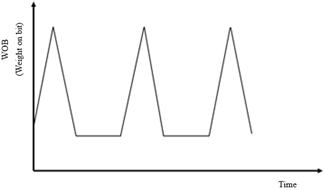Figure 1. Intermittently pulsating impact load

By contrast, the flexible impact PDM produces an axial force that changes like an alternating sine (cosine) curve. The resulting load increases and decreases periodically with time (Figure 2). The flexible alternating load of the flexible impact PDM could effectively extend the service life of components.

## 2.png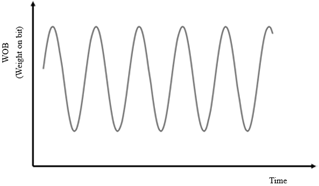2.1 Control system and working principle of hydraulic oscillator

During the surface drilling of horizontal wells or extended reach wells, the drilling pipes will cling to the wall of the well under the action of gravity. The large frictional force between the drilling pipes and the wall prevents the effective application of bit weight on the drill bit, thus affecting the ROP and depth. To improve the ROP and depth, it is highly necessary to reduce the friction between the drilling tool and the well wall. The hydraulic oscillator can effectively lower this friction, ensuring that the changing bit weight can be transmitted to the drill bit in an effective and timely manner [12-14].

As shown in Figure 3, the typical hydraulic oscillator consists of a vibration part, a power part and a disc valve part from top to bottom. Among them, the power part is a 1:2-lobe PDM connected to the moving valve block at the bottom. After the flushing liquid enters the PDM, the rotor starts to rotate and drives the lower valve block to reciprocate on a plane (Figures 4 and 5), leading to periodical changes of the flow area.

## 3.png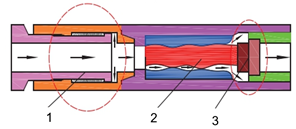1.Vibration part; 2. Power part; 3. Disc valve part

Figure 3. Structure of a hydraulic oscillator manufactured by National Oilwell Varco (NOV)

When the flow area reaches the minimum, pressure will build up in the upper part, forcing the disc spring in the vibration part to compress and accumulate energy. When the flow area widens, the pressure of the vibration part and the power part will be vented, and the disc spring will return to the original shape, releasing the accumulated energy. In this way, the drilling tool assembly on the upper part will vibrate downward, turning the sliding friction into rolling friction [15-18].

## 4.pngFigure 4. The pulsating pressure

## 5.pngFigure 5. The 1:2-lobe PDM

2.2 Control system and working principle of flexible impact PDM

2.2.1 Design ideas

From the structure of the hydraulic oscillator, it can be seen that the upper end (inflow end) of the rotor, which drives the oscillator, is in a free state, while the lower end (outflow end) of the rotor is connected to the moving valve block. In a regular PDM, the upper end of the rotor is also in a free state, while the lower end is connected to a universal shaft to transmit torque.

Therefore, the two free ends of the hydraulic oscillator (the upper end of the rotor) and the PDM (the lower end of the rotor) were combined, so that the rotor is connected to a universal shaft at one end and to the moving valve block at the other end.

2.2.2 Structural plan

Based on the working methods and principles of hydraulic oscillator and the PDM, the power part and disc valve part were switched and then connected to the vibration part, that is, the structure was changed from “vibration part + power part + disc valve part (moving valve block on top)” into “vibration part + disc valve part (moving valve block on bottom) + power part”. Next, the adjusted structure was connected to a universal shaft, forming a PDM with flexible axial impact (Figure 6).

2.2.3 Working principles

After passing through the vibration part (piston structure), the flushing liquid flows through the fixed valve block, and enters the cavity between the stator and rotor via the moving valve block. Under the pressure difference, the rotor of the PDM starts to rotate, and drives the moving valve block on its top to rotate. Then, the flow area between the moving and fixed valve blocks will change periodically, producing a periodic pulse vibration. Then, flexible axial impact will be generated at the bit to break the rock with rotary impact.

## 6.png1.Vibration part; 2. Disc valve part; 3. Rotor and stator; 4. Universal shaft; 5. Transmission shaft; 6. Bit

Figure 6. Structure of flexible impact PDM

The control system of the flexible impact PDM involves the following design parameters: impact frequency, fluctuating pressure difference, impact force, piston specifications in the vibration part, and the orifice diameter of the disc valve part .

3. Planetary Motions of the PDM

3.1 Motions of rotor and stator

In the hydraulic oscillator, the number of lobes on the rotor to that on the rotor is 1:2. During the operation, the special structure makes the rotor reciprocate in the radial direction. However, the lobe ratio cannot produce a sufficiently large torque for the PDC bit to break rocks.

In fact, underground hard rocks are often drilled with stage 4 Ф73 mm 7:8-lobe PDM or stage 3 Ф89 mm 5:6-lobe PDM. Unlike the 1:2-lobe PDM, the rotor of these two PDMs will not reciprocate in the radial direction. Thus, the motion law of the PDM rotor should be explored to facilitate the subsequent analysis.

According to the kinematics theory, the planar planetary motions of the rotor and stator of the PDM can be regarded as the relative pure rolling of the two instantaneous circles. Hence, the rolling-in method (Figure 7) was adopted to analyze these motions .

## 7.pngFigure 7. Rolling-in method

As shown in Figure 7, the rotor (the inner circle) rolls along the outer circle formed by the stator, while rotating about O' in the clockwise direction by the angle $\frac{\varphi}{n}$. In the meantime, O' makes a circular motion of radius e about center O of the fixed coordinate system, that is, the rotor revolves around O with an eccentricity e in the counterclockwise direction by the angle $\frac{N \varphi}{n}$. Therefore, the revolution angle is always numerically N times the rotation angle, with N being the number of lobes of the rotor. In other words, the revolution-rotation speed ratios of single-lobe rotor and five-lobe rotor are 1:1 and 5:1, respectively, and the rotor’s revolution speed is numerically equal to the impact frequency of the flexible impact PDM .

3.2 Motions of the disc valve part

The disc valve part is composed of a fixed valve block and a moving valve block. Each valve block has an orifice with diameter r. The orifice of the fixed valve block features an eccentricity e. As shown in Figure 8, the flushing liquid flows into the power part to sustain the normal operation of the PDM, while the planetary motions of the rotor drive the continuous operation of the two valve blocks, leading to constant changes in the flow area .

## 8.pngFigure 8. Fixed and moving valve blocks

## 9.png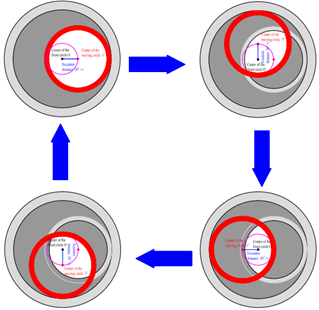Figure 9. Time variation in flow area

Figure 9 illustrates the time variation of the flow area on the moving valve block, under the effect of the rotor. The changing flow area alters the speed of the liquid flow, which in turn changes the inner pressure, producing a fluctuating pressure difference. Since the axial impact force is essentially the product between the fluctuating pressure difference (Δp) and the stressed area (S) of the piston, fluctuating pressure difference produces a water hammer phenomenon, forming a flexible axial impact.

Considering the fluctuating pressure difference induced by the changing flow area, it is necessary to probe into the flow area (A) before exploring the fluctuating pressure difference (Δp). Let r be the radius of orifice, O1 be the center of the flow area, O be the center of the fixed circle, and O2 be the center of the moving valve block, which makes a circular motion about O with the radius e. Then, the revolution speed of the moving valve block equals the speed of the PDM rotor ω.

At any moment, the distance between O1 and O2 can be expressed as:

$2 \mathrm{L}=2 \mathrm{e}|\sin (\omega t / 2)|$     (1)

$\mathrm{L}=\mathrm{e}|\sin (\omega t / 2)|$     (2)

Then, the area of the triangle whose vertices are the centers of the three circles can be calculated by:

$A 1=\operatorname{Lr} \sin \theta$     (3)

$\theta=\cos ^{-1} L / r$     (4)

The sum of the fan-shaped areas can be obtained as:

$\mathrm{A} 2=\theta r^{2}$     (5)

Therefore, the time variation of the flow area (the blank area) can be described as:

$\mathrm{A}=2(\mathrm{A} 2-\mathrm{A} 1)$      (6)

$\mathrm{A}=2\left(\theta r^{2}-\mathrm{Lr} \sin \theta\right)$     (7)

$A=2\left(r^{2} \cos ^{-1}\left(\frac{e\left|\sin \left(\frac{\omega t}{2}\right)\right|}{r}\right)\right)$

$-\operatorname{er}\left|\sin \left(\frac{\omega t}{2}\right)\right| \sin \left(\cos ^{-1}\left(\frac{e\left|\sin \left(\frac{\omega t}{2}\right)\right|}{r}\right)\right)$     (8)

4. Calculation of Hydraulic Parameters

As shown in Figure 10, the hydraulic parameters of the flexible impact PDM for underground directional drilling in coalmines were calculated in the following steps:

Step 1. The PDM and mud pump model were selected based on the diameter of drill holes. The mud pump should produce the highest possible pressure, realize stable displacement, and satisfy the normal working requirements of the PDM.

Step 2. The fluctuating pressure difference was empirically set as 10-15% of the highest pressure of the mud pump. This value could be properly increased for extremely hard rocks. Note that an excessively high fluctuating pressure difference will damage pump head, the PDM, and other accessories.

Step 3. The piston parameters were calculated by three principles: (1) The piston should have a reasonable stressed area; if the area is too large, the orifice will be narrowed, pushing up the flow rate and pump pressure; if the area is too small, the axial impact force will be insufficient to break the rocks. (2) The strength of the vibration part must be guaranteed; the walls of each component in this part should not be smaller than the thinnest thickness of the PDM. (3) The axial impact force should be calculated by F=Δp×S, and optimized based on the actual working conditions.

Step 4. Since the flow area of the disc valve depends on the eccentricity e, the orifice diameter was calculated based on the fluctuating pressure difference, which was determined based on the eccentric distance between the stator and rotor of the target PDM.

Step 5. The impact frequency was computed based on the displacement per revolution of the target PDM, and the revolution-rotation speed ratio.

## 10.pngFigure 10. Determination of hydraulic parameters of the flexible impact PDM

Table 1. Performance parameters of Ф95mm 5:6-lobe PDM

 Model Outer diameter mm Lobe ratio Number of stages Displacement L/min Rotation speed r/min 5LZ95×7.0-3 95 5:6 3 320-800 124-300 Working pressure drop MPa Output torque N·m Maximum pressure drop MPa Maximum torque N·m Maximum bit weight kN Output power kW 2.4 833 3.39 1177 55 32
5. Example Analysis

Considering the drill hole diameter (Ф120mm) and drilling tool grading for hard rocks, this section attempts to calculate the hydraulic parameters of a Ф95 mm 5:6-lobe flexible impact PDM (Table 1). The mud pump was of the model BLY460, with a designed displacement of 390 L/min (6.5 L/s).

5.1 Setting of axial impact force

As mentioned above, the axial impact force can be calculated by:

$F=\Delta p \times S$     (9)

where, Δp is the fluctuating pressure difference (MPa); S is the piston area (m2).

(1) Determining the pressure difference

Eq. (9) shows that the axial impact force depends on fluctuating pressure difference and piston area. The greater the fluctuating pressure difference, the larger the impact force. In actual construction, the BLY460 mud pump can only create a pump pressure of 11MPa, below the highest possible level of 13MPa. Hence, the fluctuating pressure difference should not be too large. Otherwise, the drill hole depth and pump life will both be affected. Based on construction experience, the fluctuating pressure difference was set to 1.5MPa to ensure the normality of drill hole depth and pump operations.

(2) Calculating the piston area

To meet the strength requirements on the piston, the outer diameter of the vibration part that contains the piston should be the same with that of the PDM (Ф95 mm), the wall thickness of this part should be the same with that at the thinnest point of the Ф95 mm PDM (10mm), and the wall thickness of the piston should not fall below 10mm. Hence, the wall thickness of the said part was set to 10mm, the wall thickness of the piston to 10mm, the diameter of the spring chamber to 10mm. Then, the remaining diameter was 35mm, including the orifice diameter. Then, the axial impact force can be calculated as:

$\mathrm{F}=1.5 \times 10^{6} \times \frac{\pi}{4} \times 35^{2} \times 10^{-6}=1.44 \mathrm{kN}$.

The obtained axial impact force is too small to effectively break the rocks. To solve the problem, the multi-stage piston design was introduced to distribute the stressed area of the conventional piston evenly to each stage. Without changing the pressure, the multi-stage design could increase the total piston area, so that the axial impact force is large enough to break the rocks.

Considering the wall thickness of each component and the water inlet size of the PDM, the inner diameter and outer diameter of the multi-stage piston were determined as Ф35 mm and Ф75 mm, respectively, and the piston structure was determined as the serial connection of three stages (Figure 11). Then, the axial impact force can be computed as:

$\mathrm{F}=1.5 \times 10^{6} \times \frac{\pi}{4}\left(75^{2}-35^{2}\right) \times 10^{-6} \times 3=15.5 \cdot \mathrm{kN}$.

The obtained axial impact force meets the theoretical requirements. Assuming that the mechanical efficiency is 80%, the actual axial impact force was about 12.4kN.

## 11.png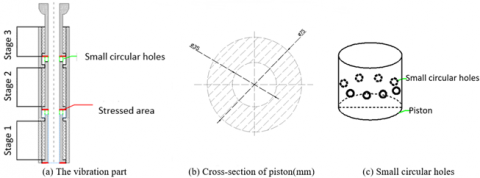Figure 11. Design of three-stage piston

5.2 Calculating orifice parameters

The periodical changes of the orifice area lead to pressure fluctuations, producing an axial impact force. According to the orifice throttling theory, the relationship between throttling pressure drop and volumetric flow rate can be expressed as:

$Q=\mu A \sqrt{\frac{2 \Delta P}{\rho}}$     (10)

Let S0 and Stbe the orifice areas corresponding to the largest and smallest fluctuating pressure difference. Then, Eq. (10) can be rewritten as:

$\Delta p=\frac{\rho Q^{2}\left(A_{\max }^{2}-A_{\min }^{2}\right)}{2 \mu^{2} A_{\max }^{2} A_{\min }^{2}}$     (11)

where, Δp is the fluctuating pressure difference (Pa); Q is the flow rate (m3/s); Amax and Amin are the maximum and minimum flow areas of the disc valve, respectively (m2); µ=1 is the valve flow coefficient; ρ=1.0×103 kg/m³ is the density of the flushing liquid.

According to Eq. (8), Amax and Amin can be respectively calculated by:

$A_{\max }=\pi r^{2}, A_{\min }=\left(\frac{\sin ^{-1} \frac{\sqrt{r^{2}-e^{2}}}{r}}{90} \pi r^{2}-2 e \sqrt{r^{2}-e^{2}}\right)$.

where, e=4.5mm is the eccentric distance between the centers of fixed and moving circles. Substituting Amax and Amin into Eq. (10), it can be obtained that r=9.2 mm, Amax=265.9 mm2, and Amin=107.1 mm2 (Table 2).

Table 2. Parameters of Ф95mm 5:6-lobe flexible impact PDM

 R (mm) e (mm) Q (m3/s) Amax(m2) Amin (m2) ρ (kg/m3) P (MPa) 6 4.5 6.5×10-3 113.10×10-6 16.29×10-6 1,000 77.9 8 4.5 6.5×10-3 201.06×10-6 65.00×10-6 1,000 4.48 9 4.5 6.5×10-3 254.47×10-6 99.41×10-6 1,000 1.81 9.1 4.5 6.5×10-3 260.16×10-6 103.21×10-6 1,000 1.67 9.2 4.5 6.5×10-3 265.90×10-6 107.08×10-6 1,000 1.54 9.3 4.5 6.5×10-3 271.72×10-6 111.01×10-6 1,000 1.43 9.4 4.5 6.5×10-3 277.59×10-6 115.00×10-6 1,000 1.32 9.5 4.5 6.5×10-3 283.53×10-6 119.06×10-6 1,000 1.23 9.6 4.5 6.5×10-3 289.53×10-6 123.18×10-6 1,000 1.14

5.3 Impact frequency

The impact frequency of the PDM is related to the revolution speed of the rotor, which is N times its rotation speed (N is the number of lobes in the rotor). The relationship between the rotation speed and the displacement of the mud pump can be described as:

$\mathrm{n}=\frac{60 Q}{q}$     (12)

Taking the displacement of 6.5L/s (390L/min), the displacement per revolution of our PDM was 2.6L/r, corresponding to the impact frequency of f=12.5Hz (Table 3).where, n is the rotation speed of the rotor (r/min); Q is the displacement (L/s); q is the displacement per revolution (L/r). The Q value only depends on the linear structure and geometric size of the PDM. For the Ф95mm 5:6-lobe flexible impact PDM, the Q value equals 2.6L/r.

Table 3. Relationship between mud pump displacement and hydraulic parameters of Ф95mm 5:6-lobe flexible impact PDM

 Mud pump displacement (L/s) Displacement per revolution (L/s) Theoretical rotation speed (r/min) Impact frequency (Hz) Pressure drop (MPa) Axial impact force (kN) 1.0 2.6 23.08 1.92 0.037 0.036 1.5 2.6 34.62 2.88 0.082 0.852 2.0 2.6 46.15 3.85 0.146 1.514 2.5 2.6 57.69 4.81 0.228 2.366 3.0 2.6 69.23 5.77 0.329 3.407 3.5 2.6 80.77 6.73 0.448 4.638 4.0 2.6 92.31 7.69 0.585 6.058 4.5 2.6 103.85 8.65 0.740 7.667 5.0 2.6 115.38 9.62 0.913 9.465 5.5 2.6 126.92 10.58 1.105 11.453 6.0 2.6 138.46 11.54 1.315 13.630 6.5 2.6 150.00 12.50 1.544 15.542 7.0 2.6 161.54 13.46 1.790 18.552 7.66 2.6 176.77 14.73 2.110 21.868

6. Simulation Verification

6.1 Modelling of backpropagation neural network (BPNN)

(1) Input layer modeling

The axial impact force is mainly affected by five parameters: mud pump displacement, displacement per revolution, theoretical rotation speed, impact frequency, and pressure drop. Therefore, the number of input layer nodes m should be 5.

(2) Hidden layer modeling

The number of hidden layer nodes must be fewer than N-1, with N being the number of training samples. Otherwise, the system error of the BPNN will have little to do with the features of the training samples, and thus tend to be zero. That is, the BPNN will have no generalization ability or value in use. Based on the number of input layer nodes m, the number of hidden layer nodes can be determined by:

$l=\log _{2}^{m}$ or $l=\sqrt{n+m}+a$     (13)

where, m, l, and n are the number of input layer nodes, hidden layer nodes, and output layer nodes, respectively; $\mathrm{a} \in[1,10]$ is an adjustable constant used to narrow down the interval of l. The l value generally falls between 4 and 13. The optimal l value is 4.

(3) Output layer modelling

The output layer needs to output the optimal axial impact force. Thus, this layer contains only one node. On this basis, the BPNN structure was established (Figure 12).

6.2 Simulation process and results

The data in the first 13 rows of Table 3 were inputted to the BPNN, which output the corresponding axial impact forces. The input samples and output samples are denoted as P and T, respectively.

After setting up the input and output samples, the weight W and threshold Q were initialized; the number of nodes in each layer was selected; the number of iterations and learning rate were configured. The error e between the target value and the actual value was compared with the requirement. If the error met the requirement, the simulation would be terminated. Otherwise, the error ei of each hidden layer node was calculated, and the error gradient was determined. Next, the weight was revised for a new epoch. These steps were repeated until the error e met the requirement. The entire process is explained in Figure 13.

## 12.png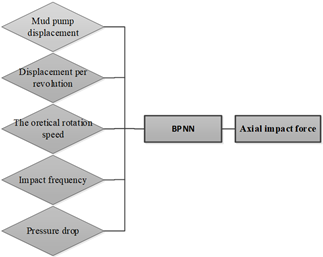Figure 12. BPNN structure

## 13.pngFigure 13. Flow of the BPNN simulation

Through repeated debugging, the BPNN parameters were optimized as follows: the number of iterations=2,000, the expected error goal=0.00000001, and the learning rate=0.01. Under these settings, the BPNN error could reach the design requirement in 662 epochs.

## 14.pngFigure 14. Curve of training error

## 15.pngFigure 15. Comparison between simulation result and test result

Table 4. Comparison between predicted values and actual values

 Sample number Actual value Predicted value Relative error 1 0.036 0.035 0.028 2 0.852 0.849 0.004 3 1.514 1.512 0.001 4 2.366 2.368 0.004 5 3.407 3.567 0.047 6 4.638 4.611 0.049 7 6.058 6.025 0.006 8 7.667 7.500 0.022 9 9.465 9.235 0.024 10 11.453 11.285 0.041 11 13.630 13.625 0.013 12 15.542 15.534 0.026 13 18.552 18.562 0.038 14 21.868 21.849 0.001

The input samples were imported to the trained BPNN. The simulation result was compared with the actual test data (Figures 14 and 15) to demonstrate the effectiveness and convergence of the BPNN.

Then, the result predicted by the BPNN was compared with the actual value to obtain the relative error:

$Relative error=\frac{\left|S_{\text {actual value }}-S_{\text {test value }}\right|}{S_{\text {atual value }}} \times 100 \%$.

As shown in Table 4 and Figure 16, the predicted axial impact force was within 5% of the actual axial impact force, i.e. the relative error was very limited.

## 16.pngFigure 16. Comparison between predicted values and actual values of axial impact force

7. Conclusions

This paper mainly aims to overcome the slow speed of underground directional drilling into hard rocks of coalmines. For this purpose, a flexible impact PDM was designed based on the structure and working principles of hydraulic oscillator and the conventional PDM. The proposed flexible impact PDM combines impact and cutting to break the rocks, while the conventional PDM solely relies on cutting in rock-breaking.

Moreover, the rotor of the proposed PDM was investigated in details, and the calculation methods were developed for the hydraulic parameters. In addition, a multi-stage piston design was formulated to overcome the limitations underground, which can produce a high axial impact force under a small pressure difference.

After that, the hydraulic parameters were calculated for Ф95mm 5:6-lobe three-stage PDM, which is suitable for drill holes of the diameter Ф120mm. The calculation shows that, when the displacement is 6.5L/s (the normal displacement underground the coalmines), the impact frequency is 12.5Hz, the fluctuating pressure difference is 1.54MPa, the impact force is 15.54kN, the inner diameter of the piston is 35mm, the outer diameter of the piston is 75mm, and the orifice radius is 9.2m. The calculated results deviate from the results of the BPNN simulation by less than 5%, indicating that the axial impact forces have been calculated accurately.

Acknowledgments

This research was supported by the National Key Research and Development Plan of China (2017YFC0808202), Major special project of science and Technology in Shanxi Province (20181101013), and Science and Technology Innovation Venture Capital Special Project of Tiandi Science and Technology Co., Ltd. (2020-TD-MS011) provided support for this work.

References

 Zhao, J.G., Zhao, J.P., Xu, C., Wu, Z.F. (2018). Composite directional drilling technology in underground coal mine. Coal Geology & Exploration, 46(4): 202-206. https://doi.org/10.3969/j.issn.1001-1986.2018.04.033

 Jian, Z.J., Zhang, Y.L., Wang, M.S., Dai, S.L. (2003). The new development of percussion-rotary drilling technique. Geology and Prospecting, 39(3): 78-83. https://doi.org/10.3969/j.issn.0495-5331.2003.03.017

 Aarsnes, U.J.F., van de Wouw, N. (2019). Effect of shock subs on self-excited vibrations in drilling systems. Journal of Petroleum Science and Engineering, 181: 106217. https://doi.org/10.1016/j.petrol.2019.106217

 Li, J., Yu, X., Zhang, Z.Q., Zhao, Z.Y. (2019). Exploring a diamond film to improve wear resistance of the hydraulic drilling impactor. Surface and Coatings Technology, 360: 297-306. https://doi.org/10.1016/j.surfcoat.2018.12.061

 Zha, C., Liu, G., Li, J., Li, Y., Xi, Y., Guo, B. (2017). Combined percussive-rotary drilling to increase rate of penetration and life of drill bit in drilling hard rock formation. Chemistry and Technology of Fuels and Oils, 53(2): 254-262. https://doi.org/10.1007/s10553-017-0802-x

 Suleimanov, R.I., Gabdrakhimov, M.S., Khabibullin, M.Y., Zaripova, L.M., Vasilyeva, E.R. (2018). The study of hydraulic hammer device in drilling tool assembly in hydraulical rotary drilling. International Journal of Engineering & Technology, 7: 28-30. https://doi.org/10.14419/ijet.v7i2.23.11877

 Wang, S.Y., Li, Q.X., Liu, J.L., Zhao, J.P., Yang, D.D. (2019). Development of impact screw motor. Coal Geology & Exploration, 47(5): 225-231. 10.3969/j.issn.1001-1986.2019.05.032

 Zhang, H., Yu, W.T., Chen, Z.S., Cai, W.J. (2014). Development of hydropulse axial-oscillation friction-reduce tool. Oil Field Machinery, 43(7): 73-76. https://doi.org/10.3969/j.issn.1001-3842.2014.07.019

 Li, B. (2014). Development and field test of hydraulic oscillator. Oil Drilling Technology, 42(1): 111-113. https://doi.org/10.3969/j.issn.1001-0890.2014.01.022

 Wang, P., Ni, H.J., Wang, R.H., Shen, Z.H., Li, Z.N., Lei, P. (2014). Journal of China University of Petroleum (Natural Science Edition), 38(4): 93-97. https://doi.org/10.3969/j.issn.1673-5005.2014.04.013

 Li, J.Y., Tan, Z.Y., Li, W., Yue, P.J. (2015). Experimental simulation of dynamic friction characteristics of interface between diamond drill and rock under impact-rotational loading. Journal of Vibration and Shock, 34(22): 210-214. https://doi.org/10.13465/j.cnki.jvs.2015.22.037

 Chen, B., Zhang, X., Liu, S. (2016). Application of hydraulic oscillator in the speed-up and efficiency enhancement of medium-deep directional well drilling. Journal of Oil and Gas Technology, 38: 66-72. http://dx.doi.org/10.12677/jogt.2016.382017

 Liu, Y., Chen, P., Ma, T., Wang, X. (2017). An evaluation method for friction-reducing performance of hydraulic oscillator. Journal of Petroleum Science and Engineering, 157: 107-116. https://doi.org/10.1016/j.petrol.2017.07.018

 Pandey, R.J., Kim, K.Y. (2018). Numerical modeling of internal flow in a fluidic oscillator. Journal of Mechanical Science and Technology, 32(3): 1041-1048. https://doi.org/10.1007/s12206-018-0205-x

 Barton, S.P., Baez, F., Alali, A. (2011). Drilling performance improvements in gas shale plays using a novel drilling agitator device. North American Unconventional Gas Conference and Exhibition, Texas, USA, pp. 886-894. https://doi.org/10.2118/144416-MS

 Kadowaki, Y. (2019). U.S. Patent No. 10,189,078. Washington, DC: U.S. Patent and Trademark Office.

 Ritto, T.G., Escalante, M.R., Sampaio, R., Rosales, M. B. (2013). Drill-string horizontal dynamics with uncertainty on the frictional force. Journal of Sound and Vibration, 332(1): 145-153. https://doi.org/10.1016/j.jsv.2012.08.007

 Xue, L., Han, H., Wang, D., Liu, X. B., Liu, H., Yu, Z.Q. (2020). Experimental study on jet pulse assembly design and numerical simulation. Petroleum Science, 17(1): 222-231. https://doi.org/10.1007/s12182-019-00396-y

 Wu, K., Ye, Z. (2019). The numerical research on rock breaking and rising mechanism of rotary-percussive drilling. Arabian Journal for Science and Engineering, 44(12): 10561-10580. https://doi.org/10.1007/s13369-019-04170-5

 Li, Z.L., Shi, B.N., Lu, J.Q., Huang, L.J. (2014). Meshing theory and application of single screw hydraulic machinery with arc tooth profile. Journal of China University of Petroleum (Edition of Natural Science), (4): 132-137. https://doi.org/10.3969/j.issn.1673-5005.2014.04.019

 Ma, G.J., Zhang, H.P., Wang, J.C. (2016). Designed and testing of the positive displacement motor driven rotary percussion drilling device. China Petroleum Machinery, 44(06): 24-27. https://doi.org/10.16082/j.cnki.issn.1001-4578.2016.06.005

 Staysko, R., Francis, B., Cote, B. (2011). Fluid hammer drives down well costs. SPE/IADC Drilling Conference and Exhibition, Amsterdam, the Netherlands. https://doi.org/10.2118/139926-MS